### Home > A2C > Chapter Ch11 > Lesson 11.1.2 > Problem11-22

11-22.
1. Convert the following degree measures to radians. Homework Help ✎

1. 45°

2. 75°

3. −15°

4. 450°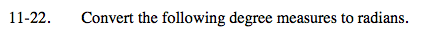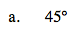$45\degree \cdot \frac{\pi }{180\degree}$

$\frac{\pi }{4}$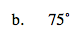See part (a).

$\frac{5\pi }{12}$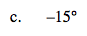See part (a).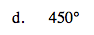See part (a).